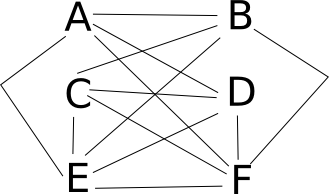Question 44

# In a six-node network, two nodes are connected to all the other nodes. Of the remaining four, each is connected to four nodes. What is the total number of links in the network?

SolutionConsider the following diagram: In this, the nodes E and F are connected to all the other nodes whereas the other four nodes are connected to four nodes each.

The total number of connections is 13.